# Can You Use a Calculator on the GMAT?Can you use a calculator on the GMAT? Yes and no. You can’t bring your own calculator to the exam, and no calculator is allowed on the GMAT Quantitative section. (This is where most students feel like they need a calculator.) However, an onscreen calculator is provided for the Integrated Reasoning section, which disappears after you finish the 12 IR questions.

## Everything You Need to Know about the GMAT Calculator

Question: But I always use a calculator for math! Should I panic about having no calculator on the GMAT?

No! Not at all!

First of all, the fact that students can’t use calculator on the GMAT places significant limits on the writers of the GMAT—they can only ask a question which could reasonably be answered without a calculator.  That saves you from having to know a whole slew of more advanced math, because they simply can’t put it on the GMAT when there’s no GMAT calculator.

Furthermore, there are some key study strategies you can implement now that will prepare you well for your no-calculator-day when you take the real GMAT.

## No GMAT Calculator: 3 Survival Tips

### Tip #1: Practice Mental Arithmetic Daily

If you were going to run a marathon six months from now, it would be a spectacularly bad idea to plan not to do any running or jogging until the day of the race.  Much like your physical muscular system, your “mental arithmetic muscles” need to be worked out and in shape for the day of the test.  Many students start with “flabby” mental math muscles because all of their lives, up until their GMAT preparation, they have been doing math with a calculator.

Of course, of course, do all your GMAT math practice questions without a calculator even in sight.  But beyond that, find little ways to do arithmetic every day.  Add up the total of your grocery order to the nearest dime while you are standing in line.  When you are driving at a certain speed, calculate how far you would drive in, say, 45 minutes at that speed.  You can even enlist a friend, spouse, sibling, or teenage child to hold a calculator, quiz your arithmetic and check your results.  See more tips in the discussion of number sense.  If you typically have done zero mental math up to this point in your life, you will be rusty at first—it will be like jogging for the first time ever.  Trust that as you practice, you will acclimate.

By the way, mental math skills will always be important in the business world: wherever there’s money, there’s numbers!

### Tip #2: Estimation without a calculator

When you have a calculator, you can do an exact calculator, but because you don’t get a calculator on the GMAT Quant, you do not need to figure out an exact answer for every math question on the GMAT: often, estimation is enough.  If you see 48.9 x 8.11, you know that’s going to be very close to 50 x 8 = 400, and that may be close enough to isolate an answer on the GMAT Problem Solving.  See the practice questions at the end for a demonstration! For more estimation-without-a-calculator strategies, read The Power of Estimation for GMAT Quant.

### Tip #3: Cancel BEFORE you multiply

This is a huge one, and nobody seems to talk about this.  If you internalize this habit, you will simply remove one of the biggest potential stumbling blocks on GMAT math.

Suppose, in the course of a GMAT math problem, you have to solve the following proportion for x: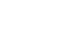Of course, the first step will be to cross-multiply:

56 × 27 = 24y

At this point in the problem, an unstrategic GMAT taker would make the disastrously bad move of finding the product of 56 x 27 (the answer is over 1000).  That’s the absolute last thing we want.  Unless someone comes into the GMAT with a gun and forces you at gunpoint to find the product of two large numbers, do not do so.

You see, the next step will be to divide by 24, to isolate y. Dividing by 24, we have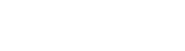At this point, we are in a perfect configuration to cancel.  We can cancel a factor of 3 from both 27 and 24, leaving 9 and 8 respectively.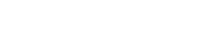Notice, instead of multiplying first, multiplying 2 two-digit numbers to get an unwieldy product greater than 1000, we left the numbers standing next to each other, unmultiplied, and waited for an opportunity to cancel.  Once the cancelling is finished, we only have to multiple 2 single-digit numbers!!  Since you don’t have a calculator on the GMAT, there will always be one way or another to avoid what looks like a large product you have to calculator.  See also the Doubling & Halving strategy.

Again, if you simply can remember to cancel before you multiply, you will be way ahead of the pack in reducing seemingly intractable calculations to grade-school easiness.

## No Calculator on GMAT: Practice Problems

1) This year, Sheila will make \$58,200.  This will be a 20% increase over her income last year.  Last year, she paid 4.8% of that year’s annual salary in taxes (federal, state, and local).  What is the dollar amount she paid in taxes?

(A) \$582
(B) \$2328
(C) \$11,640
(D) \$16,296
(E) \$27,936

2) Two small area rugs have the same area.  The first is a square, 36 inches on each side.  The second is a rectangle 24 inches wide.  What must be the length of the rectangular rug?

(A) 36
(B) 40
(C) 48
(D) 52.5
(E) 54

3) A gigantic municipal retaining wall is 38.7 feet tall by 1178 feet long.  The side that faces the city needed to be repainted.  A single gallon of specialty paint will cover 296 sq ft of the surface. How many gallons will need to be purchased to paint this wall?

(A) 73
(B) 112
(C) 155
(D) 215
(E) 497

## Can you use a calculator on the GMAT? A Summary

Can you use a calculator on the GMAT Quantitative section?  No.  There is absolutely no calculator on the GMAT Quant.  Nevertheless, this is not necessarily bad news, because it places limits on both test taker and test writer.  If between now and your GMAT you assiduously practice mental math, estimation, canceling before multiplying, and the other mental math strategies, you will be well prepared for doing GMAT math without a calculator.  Keep in mind that Magoosh has a series of lessons on mental math, as well as lessons on all the other math concepts you will need to excel on test day!## Practice Problem Explanations

1) Here, the exact numerical calculations would be ugly, and the answer choices are nicely spread out.  This problem is simply screaming for solution by estimation.  Estimate Sheila’s salary this year as \$60,000.

120% of last year = \$60000

Divide by 6

20% of last year = \$10000

Now, multiply by 5 to get 100%.

100% of last year = \$50000

Now, estimate the percentage of her taxes as 5%

5% of \$50000 = 0.05 × \$50000 = \$2500

The only answer that is anywhere near that is answer choice (B).

2) The square rug has an area of 36 × 36, and the rectangular rug has an area of 24*L, where L is the unknown length we need to find.  The areas are equal, so we have

36 × 36 = 24*L

Keeping our principle in mind, we will not find the product of 36 × 36, unless threatened at gunpoint.  Divide by 24 to isolate L.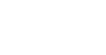Cancel a factor of 12 from the 24 and one of the 36’s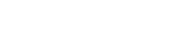Now, cancel the factor of 2 in the denominator with the other 36, and everything simplifies.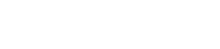3) This is a BIG wall, and again, the problem is crying out for estimation.  Let’s estimate the dimensions of this wall as 40 ft by 1200 ft.  The area would be 40 x 1200 sq ft; again, no reason to multiply those, because we know that this product has to be divided by something.  Estimate that a single gallon of paint will cover 300 sq ft.  The number of gallons needed would be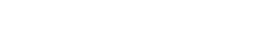Divide the 300 into 1200—it goes into it four times.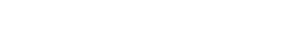•